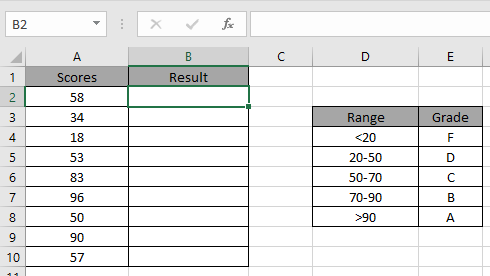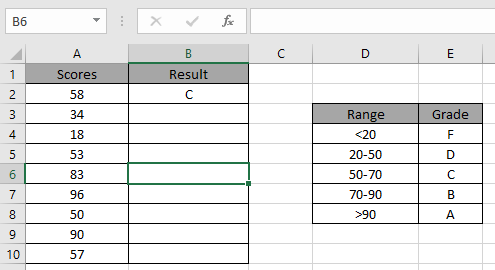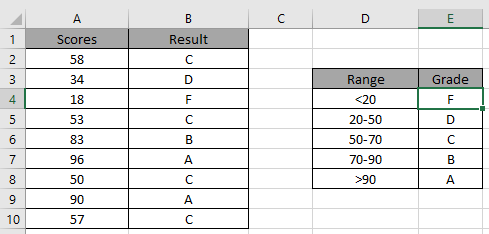# How to use the IFS Function in Excel 2019

In this article, we will learn about how to use IFS function in excel 2019. This function doesn’t work in Excel 2016 and lower versions.

IFS function is a function that runs on a logic_test like IF function. It runs multiple logic_test in one formula exactly like nested IF. It is easier to understand.
Syntax:

=IFS (test1, value1, [test2, value2], ...)

Let’s understand this function using it an example.We need to find the Grade for the corresponding Scores.
Use the Formula

=IFS(A2<20,”F”, A2<50,”D”, A2<70,”C”, A2<90,”B”, A2>90,”A”)

A2 “F”: return F if test1 satisfies.
A2 “D”: return D if test2 satisfies.
A2 ”B”: return B if test3 satisfies.
A2>90: test4
“A”: return A if test3 satisfies.Applying the formula in other cells using CTRL + D.As you can see its easy to get the Grades having multiple conditions.

Hope you understood how to use IFS function in Excel. Explore more articles on Excel logic_test here. Please feel free to state your queries in the comment box below.

Related Articles:

How to Use Nested IF Function in Excel

The Excel IF Function

IF function with Wildcards

Popular Articles:

50 Excel Shortcuts to Increase Your Productivity

How to use the VLOOKUP Function in Excel

How to use the COUNTIF function in Excel 2016

How to Use SUMIF Function in Excel

Terms and Conditions of use

The applications/code on this site are distributed as is and without warranties or liability. In no event shall the owner of the copyrights, or the authors of the applications/code be liable for any loss of profit, any problems or any damage resulting from the use or evaluation of the applications/code.